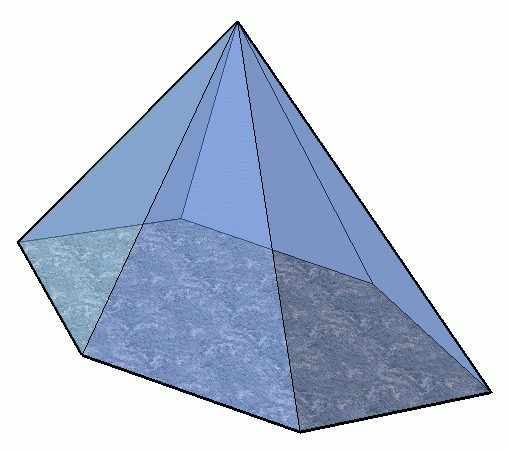SEARCH HOMEMath Central Quandaries & QueriesQuestion from Tracey, a student: How many faces, edges, and vertices does a hexagonal pyramid have? ThanksHi Tracey. Here is a hexagonal pyramid:The number of faces = the number of flat surfaces.

The number of edges = the number of lines.

The number of vertices = the number of corners.

Stephen La Rocque. >Math Central is supported by the University of Regina and The Pacific Institute for the Mathematical Sciences.